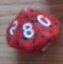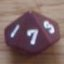Combining Two Dice Pools

## Combining Two Dice Pools

 Thu Aug 4 04:00:49 2016   by   Weylin Stormcrowe I am working on a system using a dice pool system and am not sure how to calculate probability.The system uses two pools of d6: One for Attribute and one for Skill. They are rolled against different set TN (Attribute is TN 6, Skill is TN 5). I have calculated the probability for each pool on its own. But i don't know how to combine them into a chart.For example Attribute 2 + Skill 2 (2d6, TN 6 + 2d6, TN 5).How do I combine these or what formula do I use in the program. Thu Aug 4 11:13:30 2016   by   Torben Something like:`A := 2d6; \ attribute poolS := 2d6; \ skill poolTA := 6; \ attribute targetTS := 5; \ skill target(count TA <= A) + (count TS <= S)` Thu Aug 4 14:56:48 2016   by   Weylin Stormcrowe Thanks very much, Torben Fri Oct 20 02:58:26 2017   by   Weylin Stormcrowe How do I expand this formula so that if using d10s for skill, a "10" counts as two successes? Fri Oct 20 10:10:29 2017   by   Torben Something like:`A := 2d6; \ attribute poolS := 2d10; \ skill poolTA := 6; \ attribute targetTS := 5; \ skill target(count TA <= A) + (count TS <= S) + (count 10 = S)`So I first count the skill dice that are over target, and then add the count of those that are equal to 10 (so these are counted twice). Fri Oct 20 19:43:03 2017   by   Weylin Stormcrowe Thank you very much, Torben